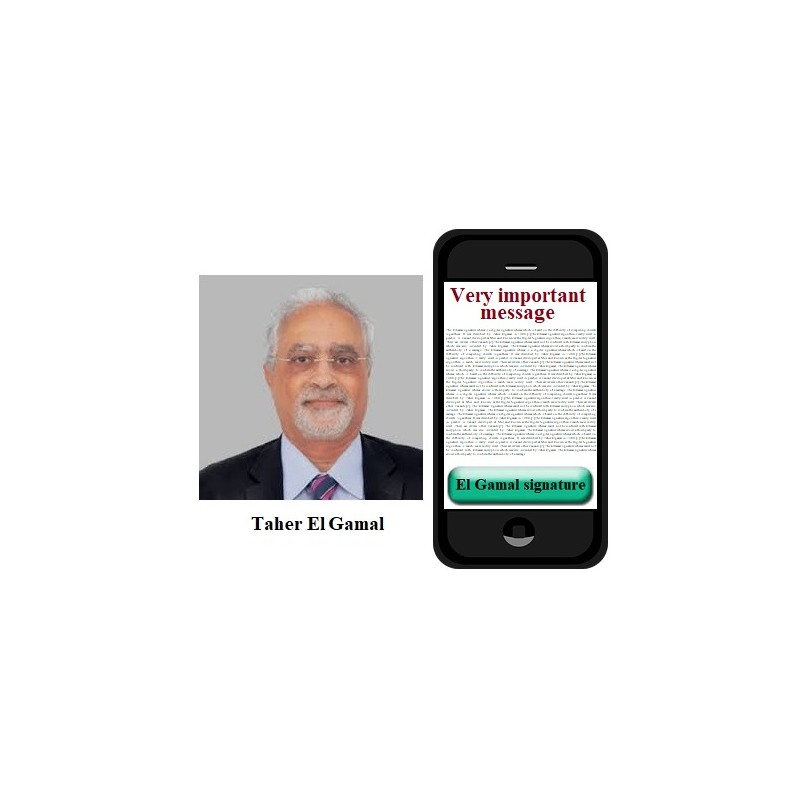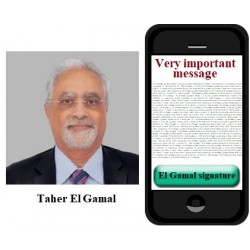•El Gamal e-signature

€3.00
Tax included

ElGamal e-signature scheme.

Quantity

COMPLETED.

This protocol is executed between Student Alice (You) and Mentor Bob. Parameter values sent by Alice are included in brackets [ ] and must be entered in the corresponding input fields.

The following functions are used in the protocol:

>> genstrongprime(bl)

>> mod_exp(g,x,p)

>> randi(z)

>> H28(D)

To compute H-function value h for the message D to be signed, i.e. H28(D)=h, it is assumed that function H28( ) is a system parameter.

1.      Generate strong prime number p of 28 bits length using Octave function

>> genstrongprime(28)

You could wait for a moment. You must check that generated p is greater than 2^27= 134217728. Send [p] to the Bob.

2.      If it is OK, find a generator g of multiplicative group Zp={1,2,3, … p-1} and send system parameters [p, g] to the Mentor.

3.      If it is OK, generate at random secret number x in the interval 1<x<p-1, compute Public Key A=gx mod p and send [A] to the Mentor.

4.      Sign message containing current date and time in the format DT=MMDDhhmm, by computing H28(D)=h.  Compute signature S=(S1, S2), consisting of two parts S1=gk mod p and S2=k-1(h-xS1) mod (p-1), where k is a random number 1<k<p-1 satisfying gcd(k, p-1)=1 and send [MMDDhhmm, S1, S2] to the Mentor for verification.

5.      Mentor’s Public Key is B, where e.g. B=….. . He sends You a signature SM=(r, s), where e.g. r=….. and s=…..  signed on his message M=….. . Verify the signature SM by computing verification parameters v1=Brrs mod p and v2=gh mod p. Send [v1, v2] to the Mentor for verification.ing prepared.

3305 Items